# 参数与变量

## 用单元格作为参数

 A B 1 3 =3*5 2 =A1*B1 3 =B2 >B2=3.14 4 =B2/2

A1是常数格，B1是表达式。A1B1的值，都可以作为参数使用，B1A2中的结果如下：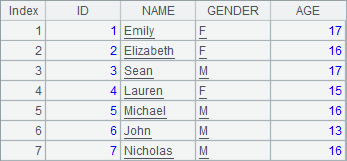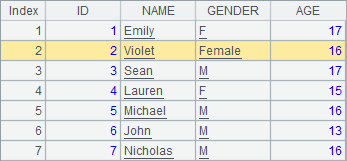B2中未设定常数，且没有表达式，这样在A3中调用B2中的格时结果为空，实际上，任何一个单元格的初始值都是null

B3B2设定了格值，此时再在A4中调用时，B2的值就已经被设定为3.14了，A4结果如下：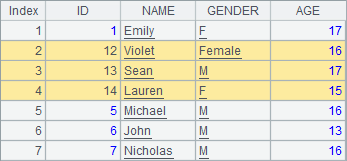A B C 1 =demo.query("select * from CITIES") 2 3 for A1 if A3.POPULATION>500000 >A2=A2+1 4 else >B2=B2+1

A3中遍历城市数据，如果城市人口大于500000，则在A2中计数，否则在B2中计数。计算后，A2B2中结果如下：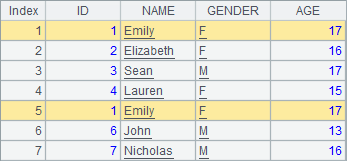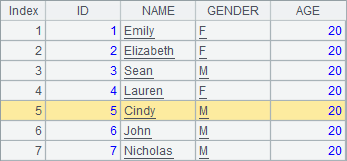## 网格参数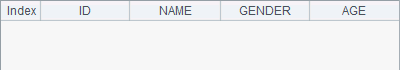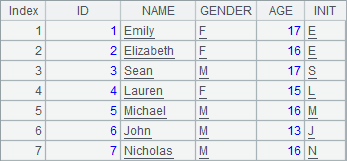A 1 =pi*4 2 =arg1+" world!" 3 =arg2.(~*~)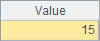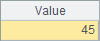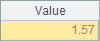A B C D 1 Big Small SAME 2 3 for num =rand(6)+1 =rand(6)+1 =rand(6)+1 4 if B3==C3 && B3==D3 >C2+=1 5 else if B3+C3+D3>10 >A2+=1 6 else >B2+=1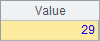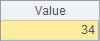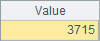## 网格变量

 A B 1 >a=5 >b=[1,3,2] 2 =a+3 =b.(~+a)

A1中和B1中分别定义了网格变量ab，这样就可以在A2B2中调用，计算后，A2B2中的结果如下：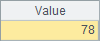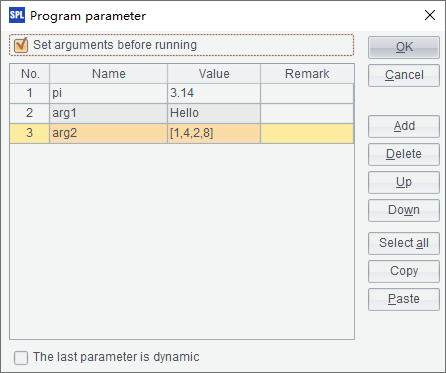A B 1 >a=5 >b=[1,3,2] 2 3 if ifv(a) >A2=b.(~+a) 4 else >B2=b

3行和第4行的代码分别准备为A2B2赋值，只有A2中有结果：B2中为空。

## 网格常量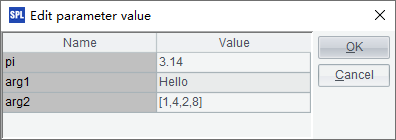A 1 =param1+" Smith" 2 =round(pi*3*3, 2) 3 =param2.sum()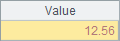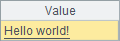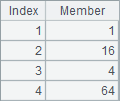## 参数、变量和常量的优先级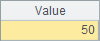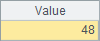A 1 =pi 2 >pi=3 3 =pi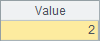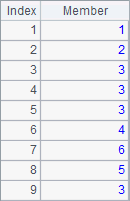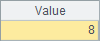A 1 2 =A1 3 >A1=3 4 =A1

A3中为"A1"赋值后，会发现单元格A1的底色变成了淡黄色，而右下角的并没有"A1"这个网格变量，说明A3实际上是在为单元格赋值。此时A1A4中的数据是相同的：